### How we do it

As a professional entity, Geoharbour Group who owns over 30 patents has completed over 300 large-scale projects over the past 20 years, total area of soil treatment has been over 10,000 hectare involving a variety of job sites, infrastructures and faciliti

# Qualification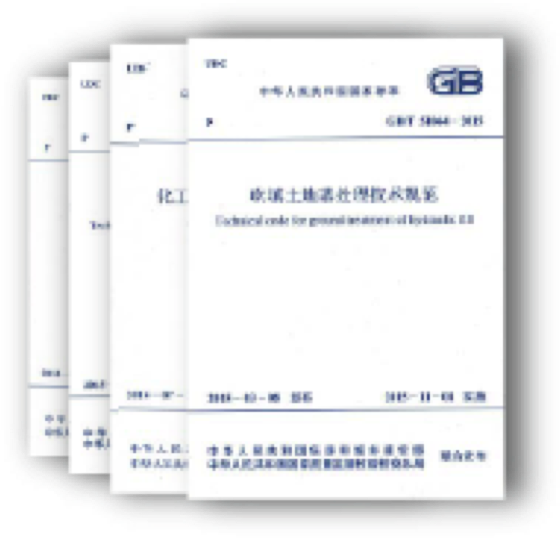The Directorate institution of the Soft Soil Improvement Technology Committee in China Editorial Unit of National Technical Code for Soft Soil Foundation and Dam Engineering in China Editorial Unit of the National Technical Code for Chemical Engineering Piperacks and Pipesleepers in China Editorial Unit of the National Technical Code for Coral Reef Foundation Treatment in China Editorial Unit of the National Technical Code for Foundation Treatment in Indonesia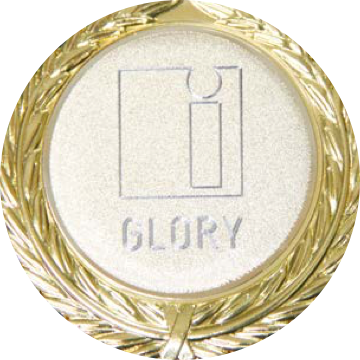The World 10th Bogsch Memory Medal

Was given to 10 honorable investors making significat contribution to the world by IFIA.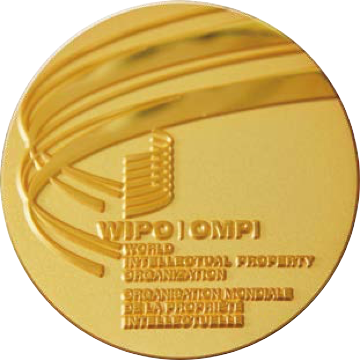The WIPO Award for Investors

Is the most important prize for investors given by WIPO.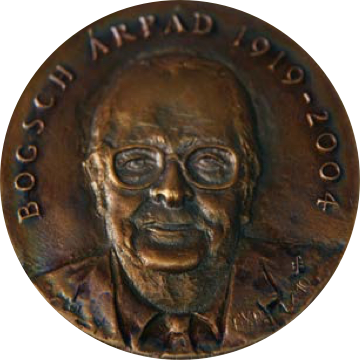The Green Invention Glory Medal

Is the most important prize for investors by World Invention Association.

ISO International Standardization--------------------------------------------------------------------------------------------------------------------------------------------------------------------------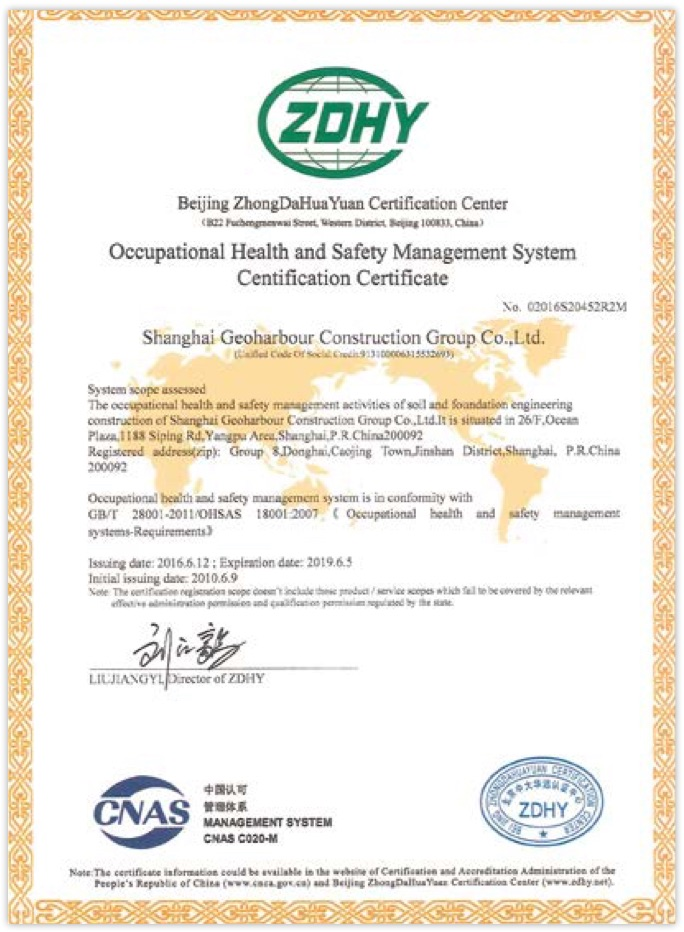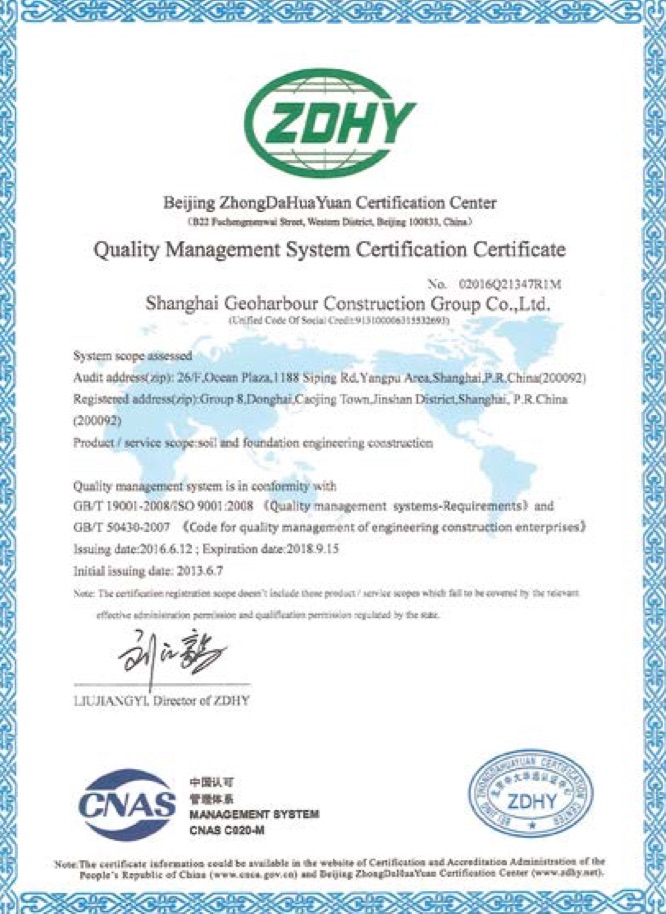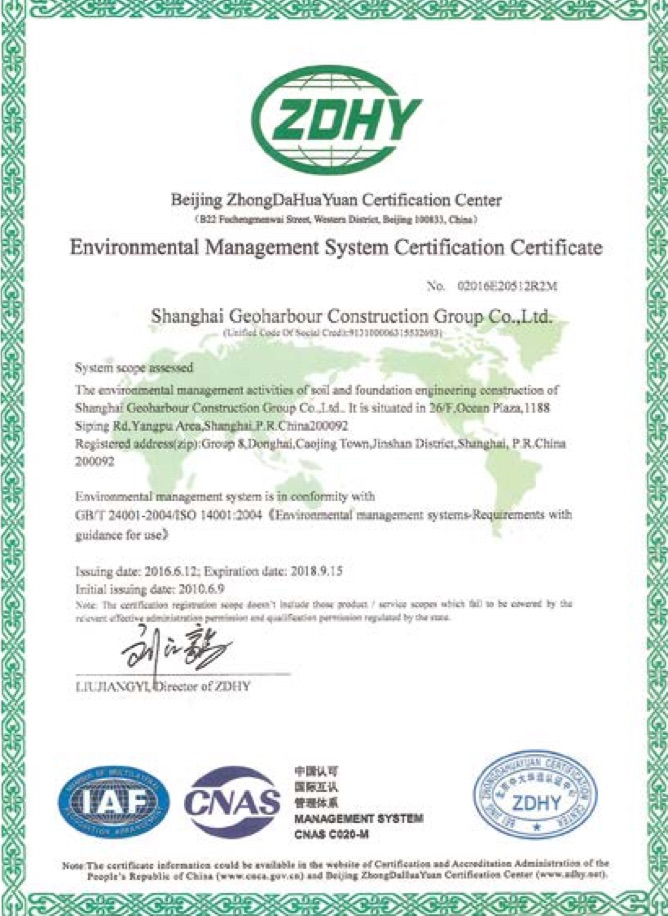Patent Registration----------------------------------------------------------------------------------------------------------------------------------------------------------------------------------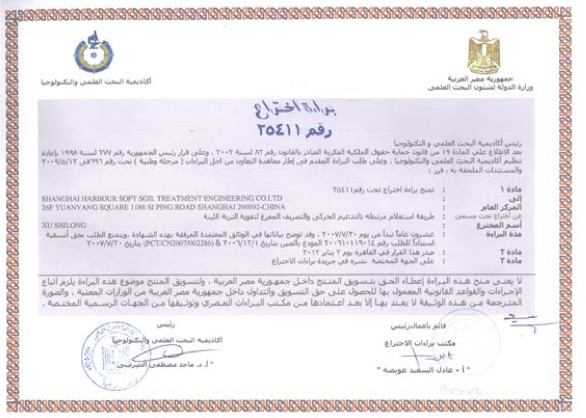Here is the patent name

Here are the details of the patent introduction The following text is a placeholder Here are the details of the patent introduction The following text is a placeholder Here are the details of the patent introduction The following text is a placeholder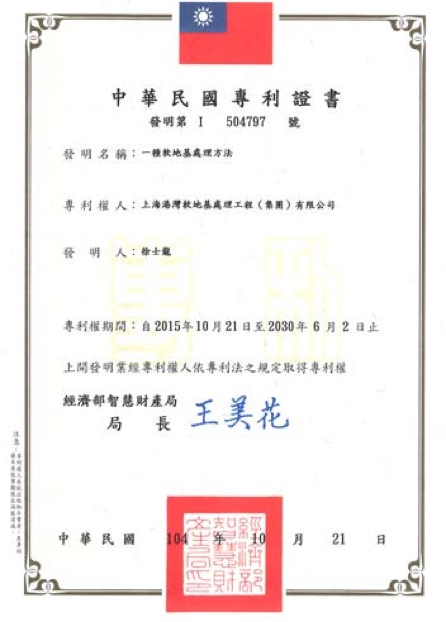Here is the patent name

Here are the details of the patent introduction The following text is a placeholder Here are the details of the patent introduction The following text is a placeholder Here are the details of the patent introduction The following text is a placeholder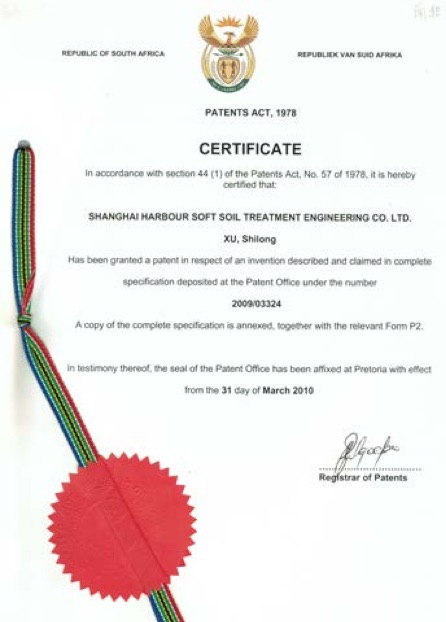Here is the patent name

Here are the details of the patent introduction The following text is a placeholder Here are the details of the patent introduction The following text is a placeholder Here are the details of the patent introduction The following text is a placeholder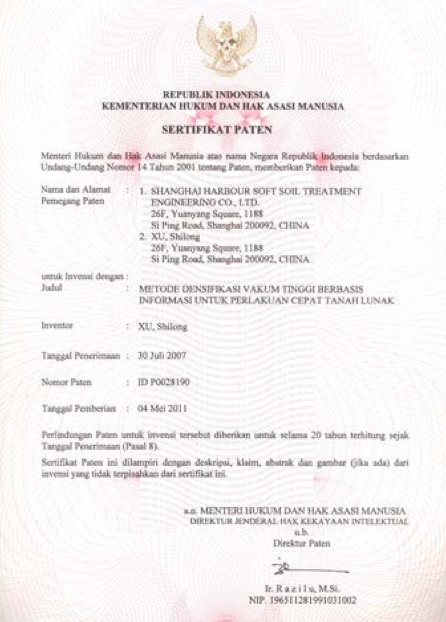Here is the patent name

Here are the details of the patent introduction The following text is a placeholder Here are the details of the patent introduction The following text is a placeholder Here are the details of the patent introduction The following text is a placeholder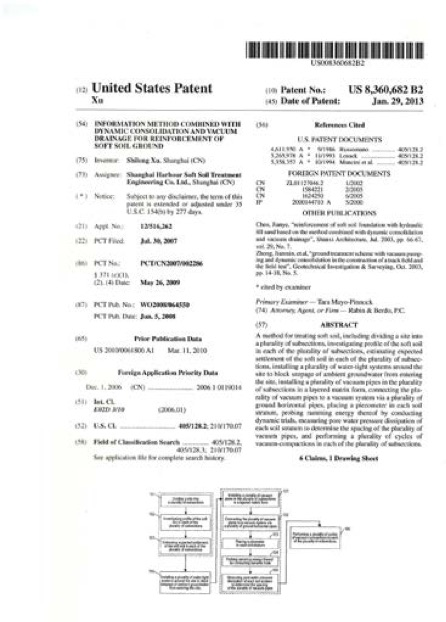Here is the patent name

Here are the details of the patent introduction The following text is a placeholder Here are the details of the patent introduction The following text is a placeholder Here are the details of the patent introduction The following text is a placeholder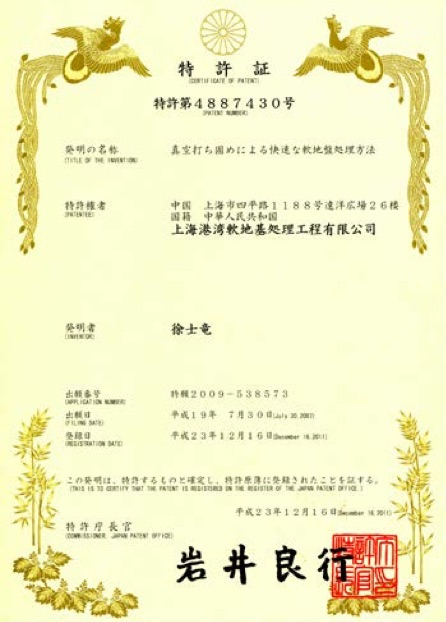Here is the patent name

Here are the details of the patent introduction The following text is a placeholder Here are the details of the patent introduction The following text is a placeholder Here are the details of the patent introduction The following text is a placeholder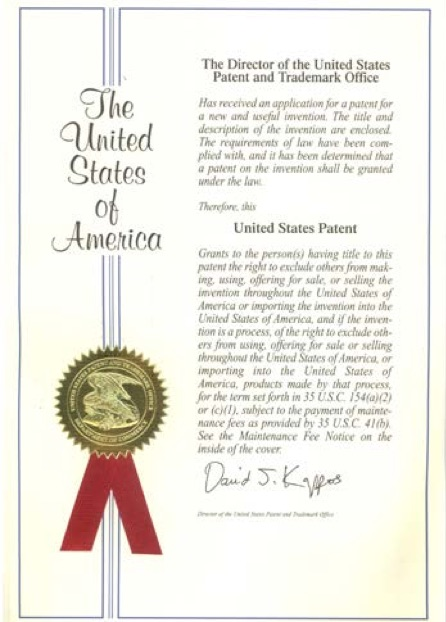Here is the patent name

Here are the details of the patent introduction The following text is a placeholder Here are the details of the patent introduction The following text is a placeholder Here are the details of the patent introduction The following text is a placeholder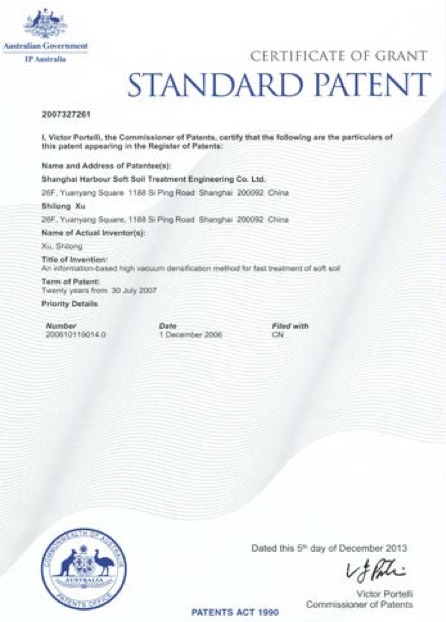Here is the patent name

Here are the details of the patent introduction The following text is a placeholder Here are the details of the patent introduction The following text is a placeholder Here are the details of the patent introduction The following text is a placeholder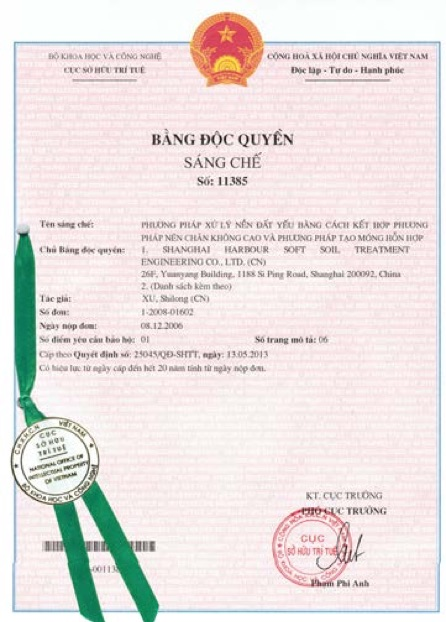Here is the patent name

Here are the details of the patent introduction The following text is a placeholder Here are the details of the patent introduction The following text is a placeholder Here are the details of the patent introduction The following text is a placeholder